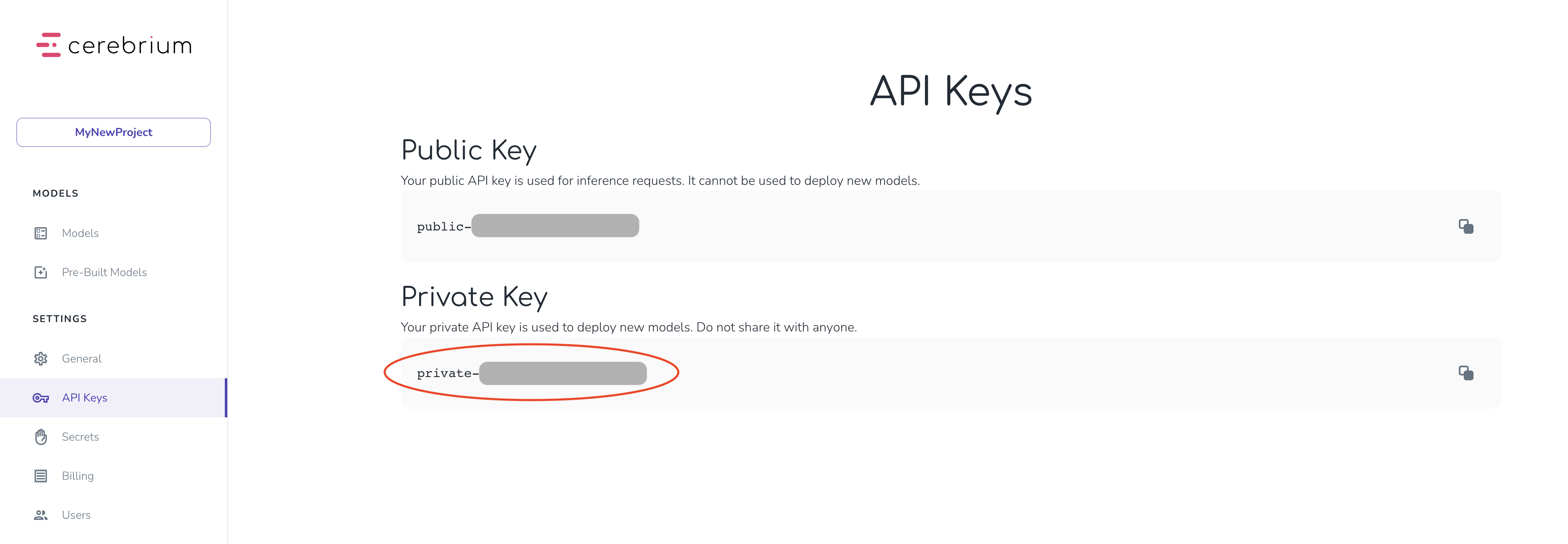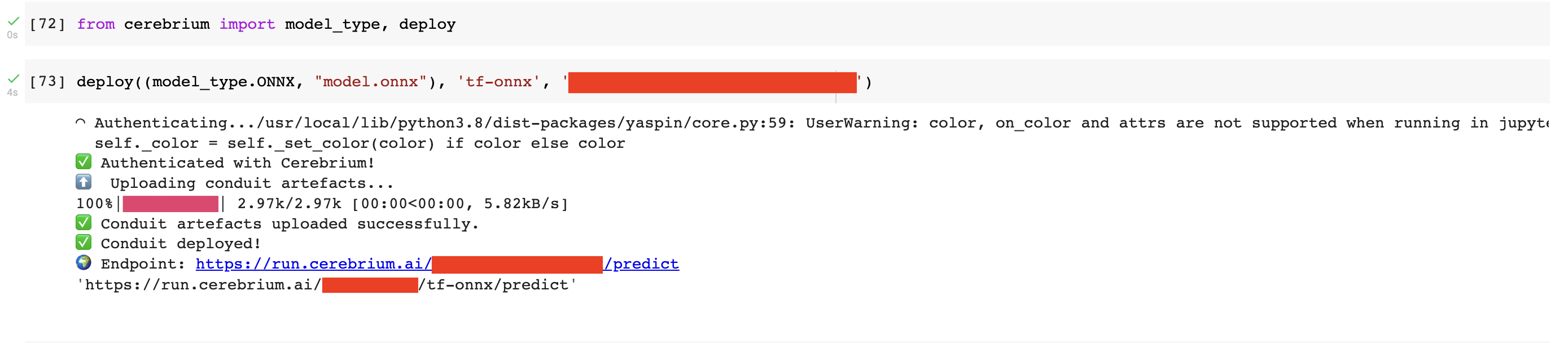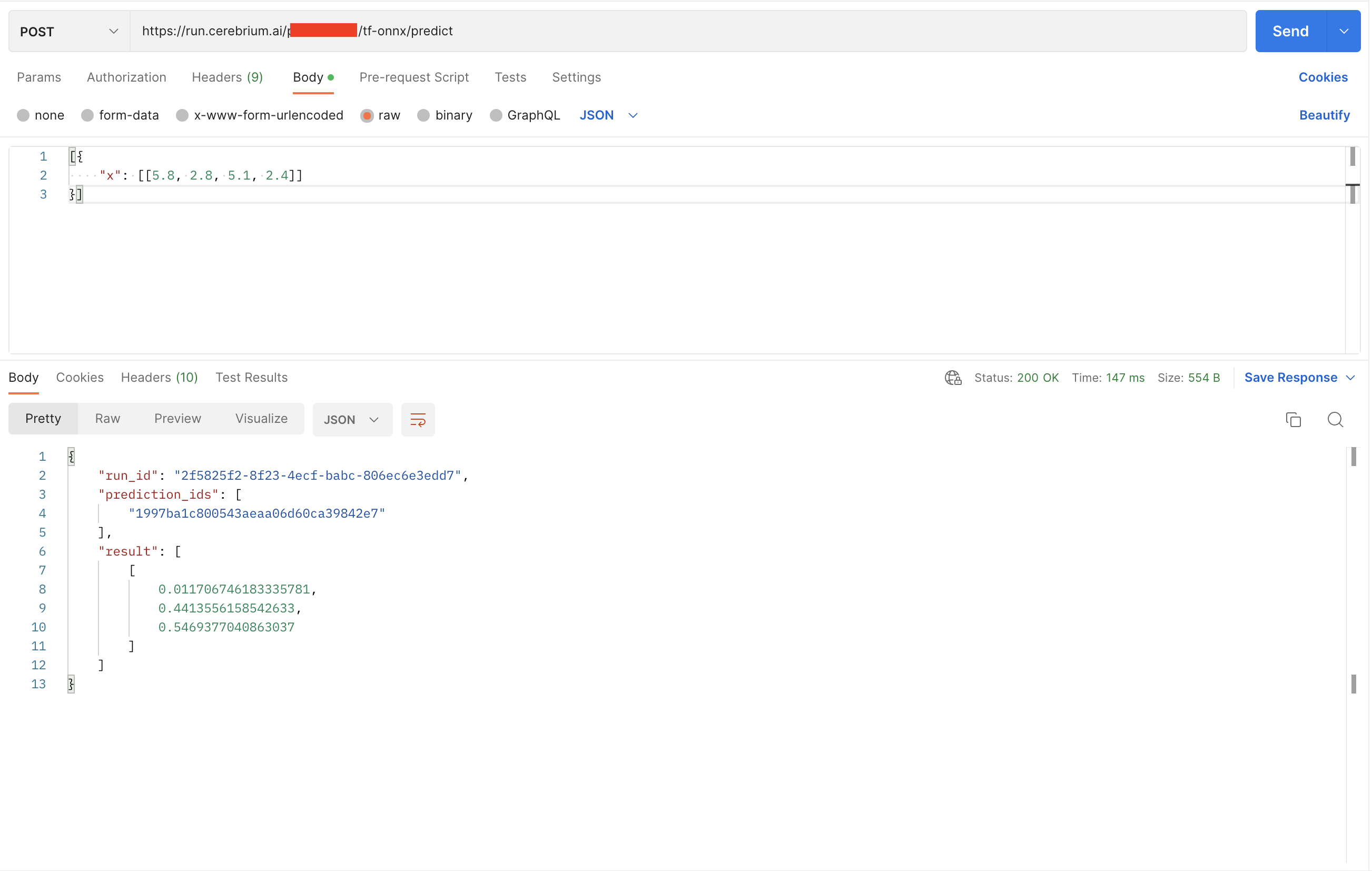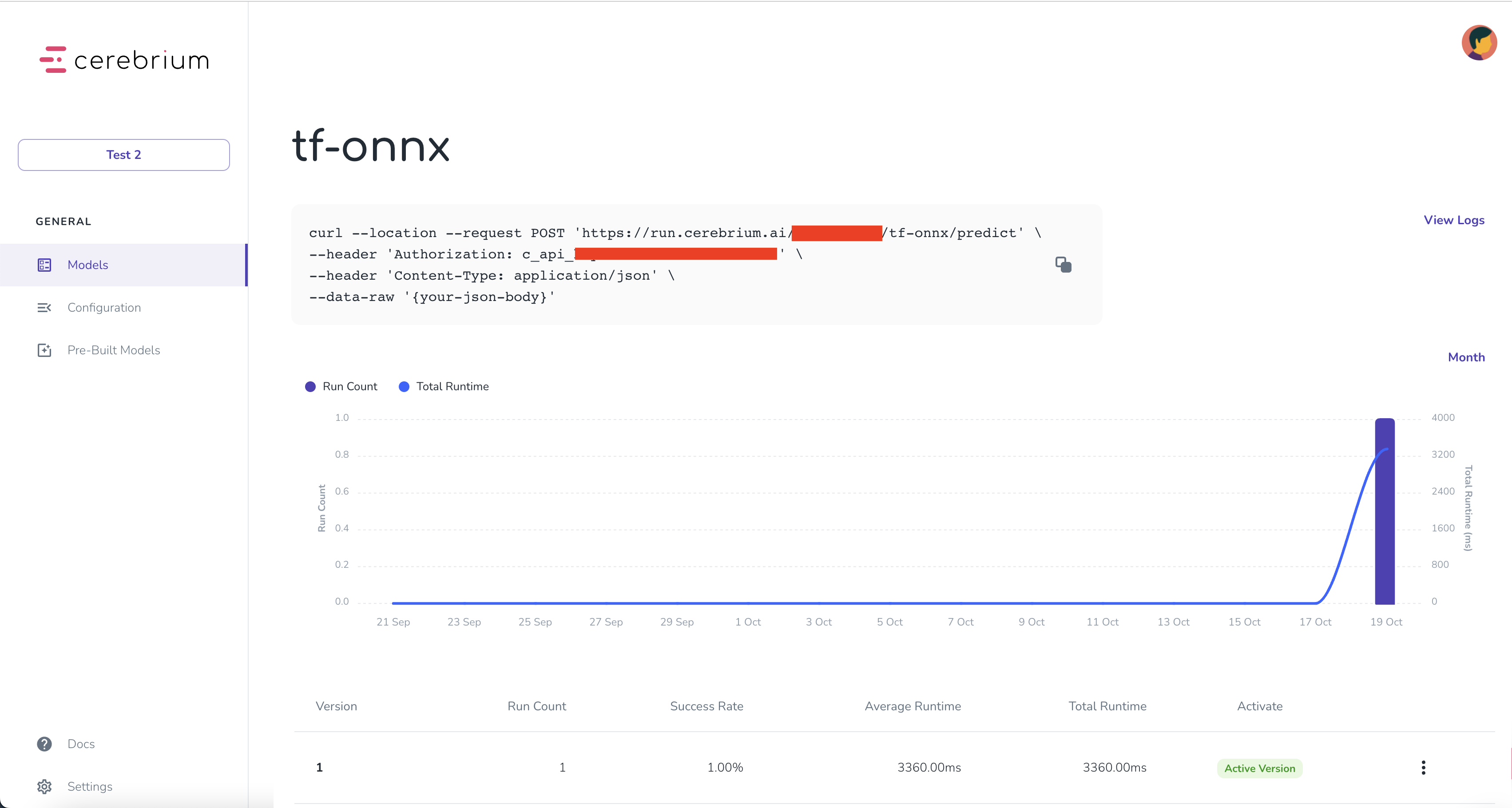## ​Intro

Cerebrium supports Tensorflow, Keras and TFLite through its support for ONNX - we will convert our model from Tensorflow to ONNX.

ONNX is an open format built to represent machine learning models. ONNX defines a common set of operators - the building blocks of machine learning and deep learning models - and a common file format to enable AI developers to use models with a variety of frameworks, tools, runtimes, and compilers.

By the end of this guide, you’ll have an API endpoint that can handle any scale of traffic by running inference on serverless CPUs/GPUs.

### ​Project set up

Before building you need to set up a Cerebrium account. This is as simple as starting a new Project in Cerebrium and copying the API key. This will be used to authenticate all calls for this project.

### ​Create a project

1. Go to dashboard.cerebrium.ai
3. Navigate to the API Keys page
4. You will need your private API key for deployments. Click the copy button to copy it to your clipboard### ​Develop model

Now navigate to where your model code is stored. This could be in a notebook or in a plain .py file.

To start, you should install the Cerebrium framework by running the following command in your notebook or terminal

pip install --upgrade cerebrium


Copy and paste our code below. This creates a simple 3-layer Neural Network that classifies flowers from the Iris dataset into 1 of 3 classes. This code could be replaced by any Tensorflow model. Make sure you have the required libraries installed.

pip install tensorflow tf2onnx onnx

import tensorflow as tf
from tensorflow.keras import layers
import pandas as pd
import numpy as np
from tensorflow.keras import datasets, layers, models
from tensorflow.keras.utils import to_categorical
from sklearn.preprocessing import LabelEncoder
from sklearn.model_selection import train_test_split

X, y = iris.data, iris.target
encoder =  LabelEncoder()
y1 = encoder.fit_transform(y)
Y = pd.get_dummies(y1).values

X_train, X_test, y_train, y_test = train_test_split(X, Y, test_size=0.2, random_state=0)

model = tf.keras.Sequential([
tf.keras.layers.Dense(10, activation='relu'),
tf.keras.layers.Dense(10, activation='relu'),
tf.keras.layers.Dense(3, activation='softmax')
])
model.compile(optimizer='rmsprop',
loss='categorical_crossentropy',
metrics=['accuracy'])

#Training
model.fit(X_train, y_train, batch_size=50, epochs=100)

#Convert to ONNX
import tf2onnx
import onnx

input_signature = [tf.TensorSpec([1, 4], tf.float32, name='x')]
onnx_model, _ = tf2onnx.convert.from_keras(model, input_signature, opset=13)
onnx.save(onnx_model, "model.onnx")


In the last 3 lines of code, you will see we:

• Define the input signature of our model. This can be a tf.TensorSpec or a numpy array defining the shape/dtype of the input. Our input for the Iris dataset is a (1,4) array, and we called the input variable ‘x’.
• Use the tf2onnx library to convert our model to the ONNX format. We define the model we would like to convert, the input signature and the opset. The opset is usually just the latest.
• Save our model to a .onnx file which is what we will use to deploy to Cerebrium.

This is all you need to deploy your model to Cerebrium! You can then import the deploy() function from the Cerebrium framework.

from cerebrium import deploy, model_type
output_flow = deploy((model_type.ONNX, "model.onnx"),"onnx-tensorflow", "<API_KEY>")Your model is now deployed and ready for inference all in under 10 seconds! Navigate to the dashboard and on the Models page, you will see your model.

You can run inference using curl

curl --location --request POST '<ENDPOINT>' \